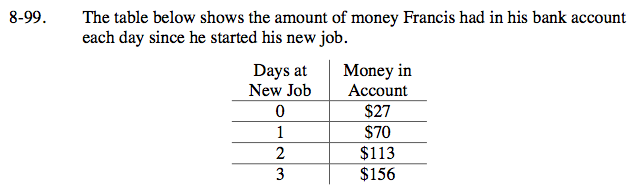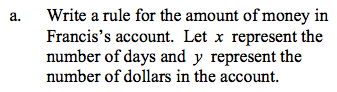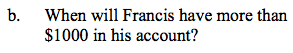Home > ACC7 > Chapter cc38 > Lesson cc38.2.3 > Problem8-99

8-99.
1. The table below shows the amount of money Francis had in his bank account each day since he started his new job. 8-99 HW eTool (Desmos). Homework Help ✎

 Days at New Job Money in Account 0 $27 1$70 2 $113 3$156
1.Write a rule for the amount of money in Francis’s account. Let x represent the number of days and y represent the number of dollars in the account.

2. When will Francis have more than \$1000 in his account?Look at the chart and see if you can determine a pattern.

y = 43x + 27Set y = 1000 and then solve the equation for x.
y = 43x + 27

Use the eTool below to help complete the problem.
Click the link at right for the full version of the eTool: CC3 8-99 HW eTool